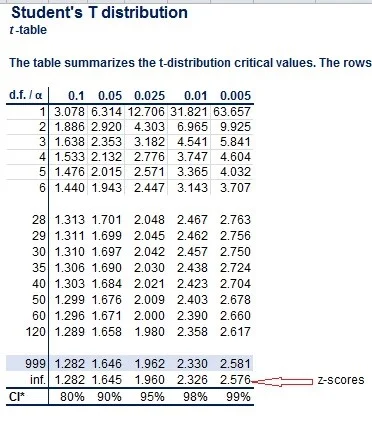06 Dec 2021

Posted on:

06 Dec 2021

0

# Z score for independent samples with unknown and different variances?

If the sample is big enough, couldn't we just circumvent the calculation for DF by using instead a Z statistic, given that the standard error here is calculated with sample variance?

Actually, we can use the z-distribution for large samples where only the sample variance is available. We can do that because of the characteristics of the t-distribution. It approaches the z-distribution as the sample size gets larger. Notice the last row values in the t-table. They are exactly the z-values at a given probability.